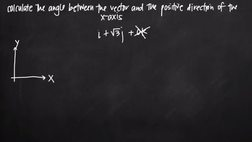PLAY PREVIEW

### Segments in this Video

#### Positive Direction of the X-axis(01:46)

FREE PREVIEW

The angle between a vector and the positive direction of the x-axis can be calculated using the xy coordinate plane.

#### Connecting Initial and Final Terminal Points(01:11)

To represent the vector i plus square root 3j, connect the initial point at the origin and the final terminal point.

#### The Tangent of the Angle Theta(00:41)

The tangent of the angle theta is equal to the length of the opposite side divided by the length of the adjacent side.

#### Replacing a Value for Theta(02:36)

Replace tangent of theta with sine of theta divided by cosine of theta equal to square root of 3.

#### Vector is in the First Quadrant(01:50)

Theta is going to be equal to pi/3, which is the same as 60 degrees. Pi/3 and 60 degrees is the only acceptable answer because the vector points toward the first quadrant.

#### Credits(00:00)

Credits

For additional digital leasing and purchase options contact a media consultant at 800-257-5126
(press option 3) or sales@films.com.

# Angle between a vector and the x-axis

Part of the Series : Integral Calc: Calculus 3
 3-Year Streaming Price: \$49.95

Share

### Description

This video tutorial works through math problems/equations that address topics in Calculus 3, Vectors. This specific tutorial addresses Angle between a vector and the x-axis.

Length: 9 minutes

Item#: BVL275649Closed Captioned